Next: Example: Up: No Title Previous: The Fundamental Theorem of

# An algebraic characterization of the solution search space: Basic Feasible Solutions

In the previous section we showed that if an LP has a (bounded) optimal solution, then it has (at least) one which corresponds to an extreme point of its feasible region. To exploit this result algorithmically, we need an algebraic characterization of the extreme point concept. This is the topic of this section.

The starting point of this discussion is the observation made at the end of the previous section, that at an extreme point, the set of binding constraints is such that it characterizes the point uniquely. Let's try to investigate what is the algebraic structure implied by this statement. Also, staying close to the general spirit of our discussion, let's examine this issue in an inductive manner.

• We know that in the 1-dim space, i.e., on the line of real numbers, a point can be identified uniquely by an equation a X = b, where.
• In the 2-dim space, a linear equation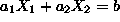defines a line, i.e., a subspace with 1 ``degree of freedom'', while the definition of a unique point requires a system of two linear equations: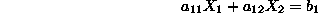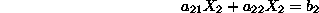with a unique solution, i.e., with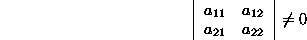Such a system of equations is characterized as linearly independent, and geometrically, it corresponds to two intersecting straight lines.

• In the 3-dim space, a linear equation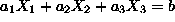corresponds to a plane perpendicular to the vector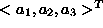. A system of two linear equations: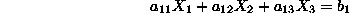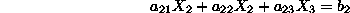for which: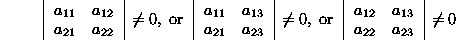corresponds to the intersection of two planes, i.e., a straight line.

Defining a unique point in the 3-dim space requires three linearly independent equations, i.e.,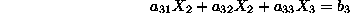with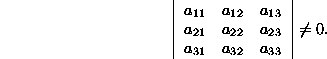• In a similar fashion, in the n-dim space, a point is uniquely defined by n linear equations which are linearly independent, i.e.,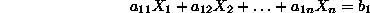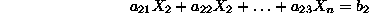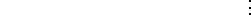with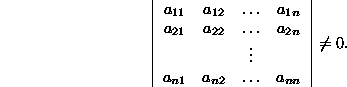Next: Example: Up: No Title Previous: The Fundamental Theorem of

UAL Data
Fri Jun 20 15:03:05 CDT 1997